# GMAT Math : Calculating the height of an equilateral triangle

## Example Questions

### Example Question #1 : Calculating The Height Of An Equilateral Triangle

If the area of an equilateral is, given a base of, what is the height of the triangle?Explanation:

We derive the height formula from the area of the triangle formula: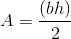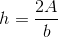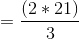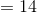### Example Question #2 : Calculating The Height Of An Equilateral Triangle

What is the height of an equilateral triangle with sidelength 20?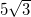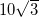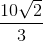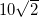Explanation:

The area of an equilateral triangle with sidelength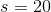is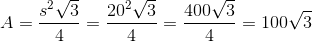Using this area forand 20 forin the general triangle formula, we can obtain: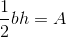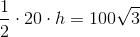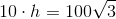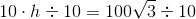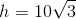### Example Question #3 : Calculating The Height Of An Equilateral Triangle

An equilateral triangle has a side length of. What is the height of the triangle?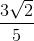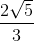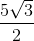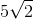Explanation:

The height of an upright equilateral triangle is the perpendicular distance from the center of its base to its top. We can imagine that this line cuts the equilateral triangle into two congruent right triangles whose height is half the length of the original base and whose hypotenuse is the original side length. In these two congruent triangles, their base, which is the height of the equilateral triangle, is the only unknown side length, so we can use the Pythagorean theorem to solve for it: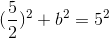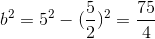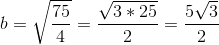### Example Question #4 : Calculating The Height Of An Equilateral Triangle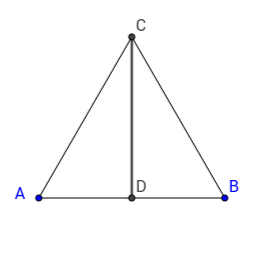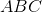is an equilateral triangle, with a side length of. What is the height of the triangle?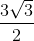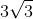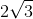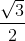Explanation:

We know the length of the side, therefore we can use the formula for the height in an equilateral triangle: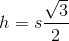, whereis the length of a side andthe length of the height.

Therefore, the final answer is.

### Example Question #5 : Calculating The Height Of An Equilateral Triangle

Given that an equilateral triangle has side lengths equal to, determine it's height in simplest form.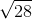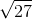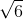Explanation:

To solve, we must use pythagorean's theorem given that we know the hypotenuse isand one side length is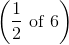. Therefore: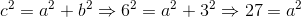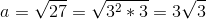Tired of practice problems?

Try live online GMAT prep today.